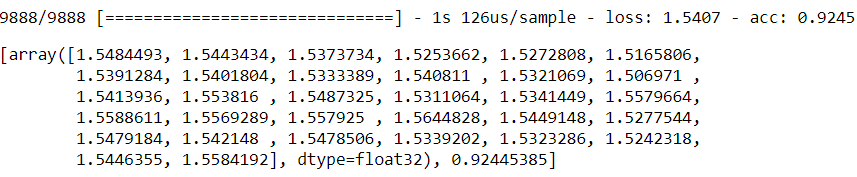# Build Differentially private Machine Learning Models Using TensorFlow Privacy

In the computer world, there are lots of data that required user wise privacy. The privacy of data and their protection are very essential for everyone. Day by day when data increases there is a possibility of privacy decreases. In this situation when more data generated required more privacy.  To solve this issue there are many different techniques to protect massive data. From the various techniques here we use one of a technique of machine learning model using TensorFlow privacy to build differential privacy model in Python.

### Differential privacy

Each individual of the group shares information of their dataset to analyze and get the required results with the assurance that their private data is secured and other people of the group access only general data this structure called differential privacy. Differential privacy used in an algorithm that shows aggregate information of group withholding the private information. For building differential privacy model TensorFlow provides the TensorFlow privacy library for the machine learning model with the privacy of training data.

### TensorFlow privacy

Tensorflow privacy model provides differential privacy mechanisms that provide privacy to sensitive training data. In some essential deep learning tasks and modeling task with data having privacy concern also in the various application which has sensitive data that can use Tensorflow Privacy. It not required any change in training in training procedure or process, it just protects privacy for training data instead of the training model. To know more about tensorflow privacy you can visit TensorFlow Privacy

## Build Differentially private Machine Learning Models Using TensorFlow Privacy in Python

In four steps we build differential private machine learning models.

Step – 1 Implementing libraries

Here, we use tensorflow_privacy, Numpy, TensorFlow libraries.

```import tensorflow as tf
from tensorflow.keras import datasets
from tensorflow.keras.utils import to_categorical
from tensorflow.keras import Sequential
from tensorflow.keras.layers import Conv2D,MaxPool2D,Flatten,Dense
from tensorflow.keras.losses import CategoricalCrossentropy
import numpy as np
from tensorflow_privacy.privacy.analysis import compute_dp_sgd_privacy
tf.compat.v1.logging.set_verbosity(tf.logging.ERROR)```

Step – 2 Reading and transforming data

```#loading the data

X_train = np.array(X_train, dtype=np.float32) / 255
X_test = np.array(X_test, dtype=np.float32) / 255

X_train = X_train.reshape(X_train.shape, 28, 28, 1)
X_test = X_test.reshape(X_test.shape, 28, 28, 1)

y_train = np.array(y_train, dtype=np.int32)
y_test = np.array(y_test, dtype=np.int32)

y_train = to_categorical(y_train, num_classes=10)
y_test = to_categorical(y_test, num_classes=10)```

Step – 3 Making model and train the model

Defining constants.

```epochs = 4
batch_size = 250```
```l2_norm_clip = 1.5
noise_multiplier = 1.2
num_microbatches = 250
learning_rate = 0.25```

Making a model by Keras.Sequential() .

```model = Sequential([
MaxPool2D(2, 1),
MaxPool2D(2, 1),
Flatten(),
Dense(32, activation='relu'),
Dense(10, activation='softmax')
])```

Defining optimizer and loss

```optimizer = DPGradientDescentGaussianOptimizer(
l2_norm_clip=l2_norm_clip,
noise_multiplier=noise_multiplier,
num_microbatches=num_microbatches,
learning_rate=learning_rate)

loss = CategoricalCrossentropy(
from_logits=True, reduction=tf.losses.Reduction.NONE)```

Compiling the model. Fitting the data into the model.

```model.compile(optimizer=optimizer, loss=loss, metrics=['accuracy'])

model.fit(X_train, y_train,
epochs=epochs,
validation_data=(X_test, y_test),
batch_size=batch_size)```

Output:Step – 4 Evaluating the model

`model.evaluate(X_test,y_test)`

Output:Computing privacy which gives epsilon and RDP order.

`compute_dp_sgd_privacy.compute_dp_sgd_privacy(n=60000, batch_size=batch_size, noise_multiplier=noise_multiplier, epochs=epochs, delta=1e-5)`

Output:From this model, we get epsilon= 0.966 and accuracy= 91% . The smaller the epsilon the more privacy guarantee. The model should have small epsilon and higher accuracy.

#### Conclusion

Here, we saw the followings:

• Differential privacy
• Tensorflow privacy
• Building differential private machine learning model